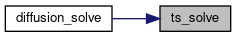XGCa
diffusion.F90 File Reference
#include "t_coeff_mod_macro.h"
#include "petsc_version_defs.h"
#include <petsc/finclude/petscts.h>
Include dependency graph for diffusion.F90: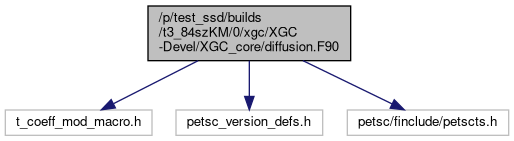## Functions/Subroutines

subroutine ts_init (a_grid, a_bc, a_ts)
Initializes the PETSc time stepper for the 2D diffusion solver. This routine sets up a system of conserving Fick's law type equations on the 2D XGC solver mesh for: density, flow and parallel and perpendicular temperature, i.e., 3*n_species+1 equations. The linear terms of the form $$\nabla \cdot \boldsymbol{D}.\nabla X$$ are discretized with linear finite elements. The nonlinear terms are discretized with finite difference. The diffusivity tensor is set up such that only trnasport perpendicular to flux-surfaces is computed by this model. More...

subroutine ts_solve (a_ts, a_dt, XX_in, blocksize)
This routine performs the actual time integration of the 2D diffusion model. More...

subroutine formrhsjacobian (ts, t_dummy, a_XX, J, Jpre, a_ts, ierr)
Update the RHS Jacobian In case the RHS Jacobian has any time dependent terms, this routine would compute the time dependent terms and put them into the final Jacobian for the present stage/step. More...

subroutine formrhsjacobianlocal (a_ts, Jpre, XX_in, nn, ierr)
Helper routine in XGC space for setting up the non-linear terms of the RHS Jacobian. This routine is used to compute any time dependent terms on the XGC solver grid. The result of this calculation will be inserted in the final RHS Jacobian. More...

subroutine rhsfunction (ts, time, a_XX, a_FF, a_ts, ierr)
Evaluates the RHS equation. More...

subroutine formrhsfunctionlocal (a_ts, time, ts_it, snes_it, XX_in, div_gamma_in, rhs_out, nn)

subroutine f_diffusion (grid, spall, psn, f0_f, f0_n, f0_df0g, df0g_tmp)
Calls the diffusion timestepper and handles the data transfer between XGC and PETSc This is the backend that is called in f_source in f0module.F90. More...

subroutine calc_deltan_gyro_avg (grid, sp, psn, delta_f_in, isp, f0_n, delta_n_out)
Computes the gyro-averaged density change due to anomalous transport for correcting the RHS of Poisson's equation in the adiabatic electron model. Takes into account the mesh-particle interpolation step exercised after all source routines are completed. More...

subroutine poly_basis_setup (grid, isp, f0_f)
Sets up a moment matrix of the current plasma distribution function and computes orthonormal polynomial basis for scaling of the plasma distribution function Polynomials: lambda_i = sum_[j=1]^4 c_[i,j] gamma_j where gamma_1 = 1, gamma_2 = v_||, gamma_3 = v_pe, gamma_4 = v_||^2, gamma_5 = v_pe^2 Inner product <lambda_i,lambda_j> = int[lambda_i lambda_j f d^3 v] Moment matrix defined by inner products <gamma_i,gamma_j> Gamma = | <1,1> <1 ,v_||> <1 ,v_pe> <1 ,v_||^2> <1 ,v_pe^2> | | <v_||,v_||> <v_||,v_pe> <v_|| ,v_||^2> <v_|| ,v_pe^2> | | <v_pe,v_pe> <v_pe ,v_||^2> <v_pe ,v_pe^2> | | <v_||^2,v_||^2> <v_||^2,v_pe^2> | | <v_pe^2,v_pe^2> | or Gamma = | <1,1> <1 ,v_||> <1 ,v_||^2> <1 ,v_pe^2> | | <v_||,v_||> <v_|| ,v_||^2> <v_|| ,v_pe^2> | | <v_||^2,v_||^2> <v_||^2,v_pe^2> | | <v_pe^2,v_pe^2> |. More...

subroutine eval_scaling_polynom (grid, den0, den, u_para, u_perp, T_para, T_perp, isp, f0_f, delta_f)
Computes the new distribution function after a diffusion time step by evaluating the scaling polynomials with the precomputed basis polynomials and the new moments (n,v_||,mu,v_||^2) More...

subroutine eval_deltaf_maxwellian (grid, dpot, den0, den, u0, u, Tpe0, Tpe, Tpa0, Tpa, isp, delta_f)

## Function/Subroutine Documentation

 subroutine calc_deltan_gyro_avg ( type(grid_type), intent(in) grid, type(species_type), intent(in) sp, type(psn_type), intent(inout) psn, real (kind=8), dimension(-f0_nvp:f0_nvp,f0_inode1:f0_inode2,0:f0_nmu,ptl_isp:ptl_nsp), intent(in) delta_f_in, integer, intent(in) isp, real(8), dimension(-f0_nvp:f0_nvp, f0_inode1:f0_inode2, f0_imu1:f0_imu2, ptl_isp:ptl_nsp), intent(in) f0_n, real (kind=8), dimension(grid%nnode), intent(out) delta_n_out )

Computes the gyro-averaged density change due to anomalous transport for correcting the RHS of Poisson's equation in the adiabatic electron model. Takes into account the mesh-particle interpolation step exercised after all source routines are completed.

Parameters
 [in] grid Grid data, type(grid_type) [in] sp Particle data, type(species_type) [in,out] psn Field data, type(psn_type) [in] delta_f_in Distribution function (v_||,v_perp), real(8) [in] isp Species index (usually isp=1 for ions), integer [in] f0_n Density distribution function [out] delta_n_out Density change of species isp after mesh-particle inter- polation and gyro-averaging

Here is the call graph for this function:subroutine eval_deltaf_maxwellian ( type(grid_type), intent(in) grid, real (kind=8), dimension(grid%nnode), intent(in) dpot, real (kind=8), dimension(f0_inode1:f0_inode2), intent(in) den0, real (kind=8), dimension(f0_inode1:f0_inode2), intent(in) den, real (kind=8), dimension(f0_inode1:f0_inode2), intent(in) u0, real (kind=8), dimension(f0_inode1:f0_inode2), intent(in) u, real (kind=8), dimension(f0_inode1:f0_inode2), intent(in) Tpe0, real (kind=8), dimension(f0_inode1:f0_inode2), intent(in) Tpe, real (kind=8), dimension(f0_inode1:f0_inode2), intent(in) Tpa0, real (kind=8), dimension(f0_inode1:f0_inode2), intent(in) Tpa, integer, intent(in) isp, real (kind=8), dimension(-f0_nvp:f0_nvp,f0_inode1:f0_inode2,0:f0_nmu), intent(out) delta_f )

Here is the call graph for this function:subroutine eval_scaling_polynom ( type(grid_type), intent(in) grid, real (kind=8), dimension(f0_inode1:f0_inode2), intent(in) den0, real (kind=8), dimension(f0_inode1:f0_inode2), intent(in) den, real (kind=8), dimension(f0_inode1:f0_inode2), intent(in) u_para, real (kind=8), dimension(f0_inode1:f0_inode2), intent(in) u_perp, real (kind=8), dimension(f0_inode1:f0_inode2), intent(in) T_para, real (kind=8), dimension(f0_inode1:f0_inode2), intent(in) T_perp, integer, intent(in) isp, real (8), dimension(-f0_nvp:f0_nvp, f0_inode1:f0_inode2, f0_imu1:f0_imu2, ptl_isp:ptl_nsp), intent(in) f0_f, real (kind=8), dimension(-f0_nvp:f0_nvp,f0_inode1:f0_inode2,0:f0_nmu), intent(out) delta_f )

Computes the new distribution function after a diffusion time step by evaluating the scaling polynomials with the precomputed basis polynomials and the new moments (n,v_||,mu,v_||^2)

Ansatz: g = sum_[i=1]^4 d_i lambda_i f ==> d_i = int(lambda_i g d^3v) / <lambda_i,lambda_i> = sum_[j=1]^4 c_[i,j] int(gamma_j g d^3v) / <lambda_i,lambda_i> After some manipulation —> g = f sum_[k=1]^4 D_k gamma_k with D_k = sum_[i,j=1]^4 int(gamma_j g d^3v * c_[i,j]*c_[i,k] / <lambda_i,lambda_i>

Parameters
 [in] grid Grid data, type(gridtype) [in] den0 Density prior to diffusion step, real(8) [in] den Density after diffusion step, real(8) [in] u_para0 Parallel flow before diffusion step, real(8) [in] u_para Parallel flow after diffusion step, real(8) [in] u_perp Perpendicular flow before/after diffusion step, real(8) [in] T0 Temperature before diffusion step, real(8) [in] T_para Para. Temperature after diffusion step, real(8) [in] T_perp Perp. Temperature after diffusion step, real(8) [in] alpha Ratio of parallel to perp temperature prior to diffusion step, real(8) [in] isp Species index, integer [out] delta_f Change of the distribution function due to anomalous diffusion, real(8)

Here is the call graph for this function:Here is the caller graph for this function:subroutine f_diffusion ( type(grid_type), intent(in) grid, type(species_type), dimension(0:ptl_nsp_max), intent(in) spall, type(psn_type), intent(inout) psn, real (8), dimension(-f0_nvp:f0_nvp, f0_inode1:f0_inode2, f0_imu1:f0_imu2, ptl_isp:ptl_nsp), intent(in) f0_f, real (8), dimension(-f0_nvp:f0_nvp, f0_inode1:f0_inode2, f0_imu1:f0_imu2, ptl_isp:ptl_nsp), intent(in) f0_n, real (8), dimension(-f0_nvp:f0_nvp, f0_inode1:f0_inode2, f0_imu1:f0_imu2, ptl_isp:ptl_nsp), intent(inout) f0_df0g, real (8), dimension(-f0_nvp:f0_nvp,f0_inode1:f0_inode2,f0_imu1:f0_imu2,ptl_isp:ptl_nsp), intent(out) df0g_tmp )

Calls the diffusion timestepper and handles the data transfer between XGC and PETSc This is the backend that is called in f_source in f0module.F90.

Parameters
 [in] grid XGC solver grid, type(grid_type) [in] spall Particle data (required for ambipolar particle flux), type(species_type) [in] psn Field data (potential, etc.), type(psn_type) [in] f0_n Density distribution function [in,out] f0_df0g Distribution function [out] df0g_tmp Temporary storage for the flux-surface avg. Maxwellian part of delta-f, real8)

Here is the call graph for this function:Here is the caller graph for this function:subroutine formrhsfunctionlocal ( type(xgc_ts) a_ts, time, ts_it, snes_it, real (kind=8), dimension(nn,a_ts%blocksize), intent(in) XX_in, real (kind=8), dimension(nn), intent(in) div_gamma_in, real (kind=8), dimension(nn,a_ts%blocksize), intent(out) rhs_out, integer nn )

Parameters
 [in,out] ts PETSc time stepping object, TS [in] time Time stamp, PETScReal, PETScReal [in] ts_it TS time step index, PETScInt [in] snes_it SNES iteration index, PETScInt [in] XX_in Input quantities [n,ui,Tipa,Tipe,{ue,Tepa,Tepe}], real(8) [in] div_gamma_in div(D.grad(n)) [out] rhs_out Nonlinear RHS terms [n,ui,Tipa,Tipe,{ue,Tepa,Tepe}], real(8) [in] nn Number of mesh vertices, integer

Here is the call graph for this function: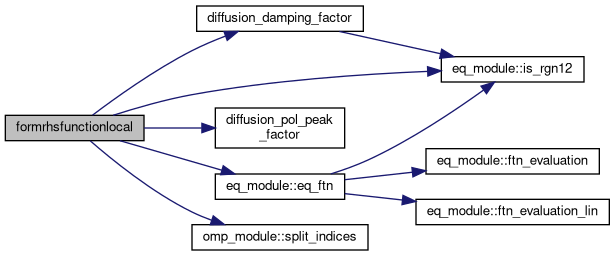Here is the caller graph for this function: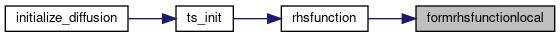subroutine formrhsjacobian ( ts, t_dummy, a_XX, J, Jpre, type(xgc_ts) a_ts, intent(out) ierr )

Update the RHS Jacobian In case the RHS Jacobian has any time dependent terms, this routine would compute the time dependent terms and put them into the final Jacobian for the present stage/step.

Parameters
 [in] ts PETSc time stepping object, TS [in] t_dummy Time stamp, PETScReal [in] a_XX Current fields, Vec [in,out] J Jacobian, Mat [in,out] Jpre Preconditioned Jacobian, Mat [in,out] a_ts XGC time stepping object, type(xgc_ts) [out] ierr Error code, PETScErrorCode

Here is the call graph for this function: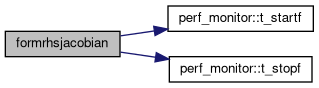Here is the caller graph for this function: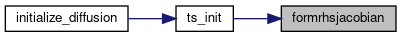subroutine formrhsjacobianlocal ( type(xgc_ts), intent(inout) a_ts, intent(inout) Jpre, real (kind=8), dimension(nn,a_ts%blocksize), intent(in) XX_in, integer nn, intent(inout) ierr )

Helper routine in XGC space for setting up the non-linear terms of the RHS Jacobian. This routine is used to compute any time dependent terms on the XGC solver grid. The result of this calculation will be inserted in the final RHS Jacobian.

Parameters
 [in,out] a_ts type(xgc_ts), XGC ts_object with grid information, etc. [in,out] Jpre PETSc Mat, the RHS Jacobian matrix [in] n real(8), perturbed electron density [in] ui real(8), A_parallel [in] Ti real(8), electrostatic potential [in] ue real(8), del^2(A_parallel) [in] Te real(8), del^2(A_parallel) [in] nn integer, mesh size [in,out] ierr PetscErrorCode
 subroutine poly_basis_setup ( type(grid_type), intent(in) grid, integer, intent(in) isp, real (8), dimension(-f0_nvp:f0_nvp, f0_inode1:f0_inode2, f0_imu1:f0_imu2, ptl_isp:ptl_nsp), intent(in) f0_f )

Sets up a moment matrix of the current plasma distribution function and computes orthonormal polynomial basis for scaling of the plasma distribution function Polynomials: lambda_i = sum_[j=1]^4 c_[i,j] gamma_j where gamma_1 = 1, gamma_2 = v_||, gamma_3 = v_pe, gamma_4 = v_||^2, gamma_5 = v_pe^2 Inner product <lambda_i,lambda_j> = int[lambda_i lambda_j f d^3 v] Moment matrix defined by inner products <gamma_i,gamma_j> Gamma = | <1,1> <1 ,v_||> <1 ,v_pe> <1 ,v_||^2> <1 ,v_pe^2> | | <v_||,v_||> <v_||,v_pe> <v_|| ,v_||^2> <v_|| ,v_pe^2> | | <v_pe,v_pe> <v_pe ,v_||^2> <v_pe ,v_pe^2> | | <v_||^2,v_||^2> <v_||^2,v_pe^2> | | <v_pe^2,v_pe^2> | or Gamma = | <1,1> <1 ,v_||> <1 ,v_||^2> <1 ,v_pe^2> | | <v_||,v_||> <v_|| ,v_||^2> <v_|| ,v_pe^2> | | <v_||^2,v_||^2> <v_||^2,v_pe^2> | | <v_pe^2,v_pe^2> |.

===> <lambda_i,lambda_j> = sum_[k,l=1]^5 c_[i,k] Gamma_[k,l] c_[j,l] ==> lambda_i^T.A.lambda_k The coefficients c_[i,l] are calculated with Gram-Schmidt process lambda_i = gamma_i - sum_[j=1]^[i-1] <gamma_i,lambda_j>/<lambda_j,lambda_j> lambda_j

The coefficients of the basis polynomials are stored in a 4x4 matrix, The inner products in the matrix A are stored in a 10-element vector starting with the main diagonal, then going through the upper side diagonals.

The moments in the matrix Gamma are evaluated from the global variable storing the distribution function –> f0_f

The coefficients c_[i,j] of the basis polynomials are stored in "diff_polynom" and the norms <lambda_i,lambda_i> are stored in "diff_polynom_norm"

Parameters
 [in] grid Grid data, type(grid_type) [in] isp Species index, integer

Here is the call graph for this function:Here is the caller graph for this function:subroutine rhsfunction ( ts, time, a_XX, a_FF, type(xgc_ts) a_ts, intent(out) ierr )

Evaluates the RHS equation.

Parameters
 [in,out] ts PETSc time stepping object, TS [in] time Time stamp, PETScReal [in] a_XX Current field data, Vec [out] a_FF RHS result vecgtor, Vec [in,out] a_ts XGC time stepping object, xgc_ts [in,out] ierr PETScErrorCode

Here is the call graph for this function: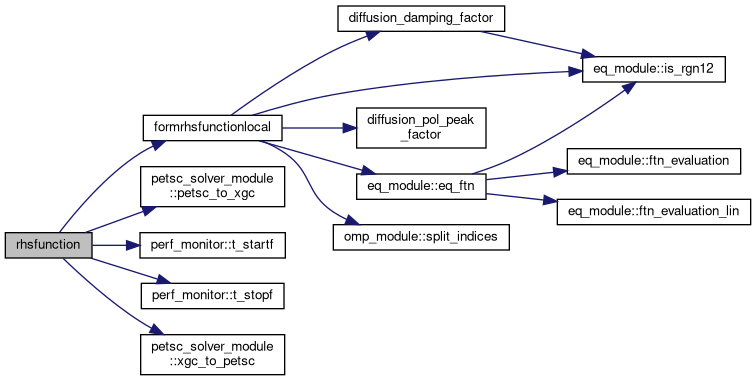Here is the caller graph for this function: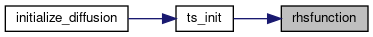subroutine ts_init ( type(grid_type), intent(in) a_grid, type(boundary2_type), intent(in) a_bc, type(xgc_ts) a_ts )

Initializes the PETSc time stepper for the 2D diffusion solver. This routine sets up a system of conserving Fick's law type equations on the 2D XGC solver mesh for: density, flow and parallel and perpendicular temperature, i.e., 3*n_species+1 equations. The linear terms of the form $$\nabla \cdot \boldsymbol{D}.\nabla X$$ are discretized with linear finite elements. The nonlinear terms are discretized with finite difference. The diffusivity tensor is set up such that only trnasport perpendicular to flux-surfaces is computed by this model.

Parameters
 [in] a_grid XGC solver grid, type(grid_type) [in] a_bc Boundary vertices for solver, type(boundary2_type) [in,out] a_ts XGC time-stepper structure, type(xgc_ts)

Here is the call graph for this function:Here is the caller graph for this function:subroutine ts_solve ( type(xgc_ts), intent(inout) a_ts, real(kind=8), intent(in) a_dt, real(kind=8), dimension(a_ts%nnode,blocksize), intent(inout) XX_in, intent(in) blocksize )

This routine performs the actual time integration of the 2D diffusion model.

Parameters
 [in,out] a_ts XGC time-stepper structure, type(xgc_ts) [in] a_dt Time step, real8 [in,out] XX_in Input moments (density, etc.), real8 [in] blocksize Number of equations, integer

Here is the call graph for this function: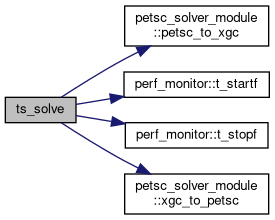Here is the caller graph for this function: